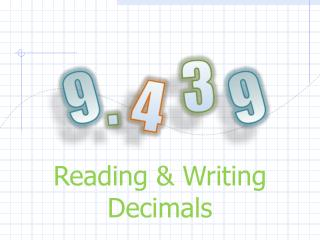DownloadDownload PresentationTélécharger la présentation- - - - - - - - - - - - - - - - - - - - - - - - - - - E N D - - - - - - - - - - - - - - - - - - - - - - - - - - -
##### Presentation Transcript

1. 3 9 9 . 4 Reading & Writing Decimals

2. Decimal Place Values Lets take a look at the places and the values of decimals. Tens Ones Tenths Hundredths Thousandths 10 .1 .01 .001 1 . Decimal

3. Reading & Writing Decimals • Say or write the number before the decimal point. • Say or write “and” when you get to the decimal point. • Say or write the number after the decimal point. • Say or write the name of the place that the decimal ends in. (tenths, hundredths, thousandths)

4. Example 8,243.67 Eight thousand, two hundred forty-three “and” sixty-seven hundredths

5. Lets Practice: • 247.017 • 16.3 • 4,764.52 • 63,920.143 and seventeen thousandths two hundred forty-seven and three tenths sixteen and fifty-two hundredths Four thousand, seven hundred sixty-four and Sixty-three thousand, nine hundred twenty . one hundred forty-three hundredths

6. From Words to Standard Form • Read everything that comes before the word “and.” Write that number down. • Place a decimal point at the word “and.” • Read the last word of the sentence to see how many decimal places you need. • Fill in the decimal places as far to the right as possible with your number, then use zeroes to fill any empty spaces.

7. Example: Write the following number in standard form:two hundred six and five thousandths 5 206 . __ __ __ 0 0 The word “thousandths” indicates that we need three decimal places. When we clean it up, the answer is 206.005

8. Lets Practice: • Six hundred fifty-three and twenty-three hundredths • Fourteen and five tenths • Three thousand, nine hundred thirty-five and sixty-one thousandths . 23 653 . 5 14 . 061 3,935

9. Expanded Form • Write the number that appears before the decimal point in expanded form. • For numbers that are decimals only, place a zero in the ones place. • Also, substitute zeroes for all spaces after the decimal point that come before the digit that you are working with.

10. Example: Write 13.361 in expanded form. Remember, deal with the number before the decimal first. + 0.3 + 0.06 + 0.001 10 + 3 Then, do the decimal part.

11. Lets Practice: • 249.617 • 16.3 • 4,764.52 200 + 40 + 9 + 0.6 + 0.01 + 0.007 10 + 6 + 0.3 4,000 + 700 + 60 + 7 + 0.5 + 0.02 .

12. Lets Practice: 200 + 40 + 9 + 0.6 + 0.01 + 0.007 249.617 16.3 4,764.52 10 + 6 + 0.3 4,000 + 700 + 60 + 7 + 0.5 + 0.02